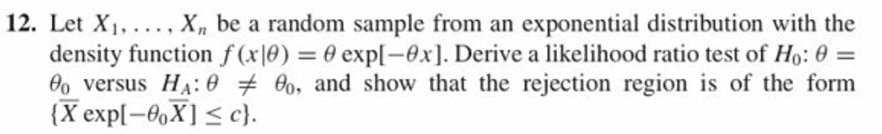# 12. Let Xi.. Xn be a random sample from an exponential distribution with thedensity function f(x10)-: θ expl-er]. Derive a likelihood ratio test of H0:0Po versus HA : θチ6, and show that the rejection region is of the form

Question

9.12 question attachedhelp_outlineImage Transcriptionclose12. Let Xi. . Xn be a random sample from an exponential distribution with the density function f(x10)-: θ expl-er]. Derive a likelihood ratio test of H0:0 Po versus HA : θチ6, and show that the rejection region is of the form fullscreen
check_circleExpert Solution
Step 1

In the given distribution function, theta is the real valued parameter specified by null hypothesis as shown below. We will test the null hypothesis based on the sample and if the null hyppthesis holds, the likelihood function would be very large at hypothesized value. This comparison happens at maximum likelihood value over the interval.

The likelihood ratio for null hypothesis vs alternative hypothesis is given by lambda which is the ratio of likelihood function at hypothesized value and the maximum likelihood over the interval. Null hypothesis is rejected if lambda is small.

Step 2

Maximum likelihood estimate...

### Want to see the full answer?

See Solution

#### Want to see this answer and more?

Solutions are written by subject experts who are available 24/7. Questions are typically answered within 1 hour*

See Solution
*Response times may vary by subject and question
Tagged in

### Statistics